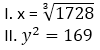SBI PO Quantitative Aptitude Quiz

Here, we are providing SBI PO Mains Study plan as there is left not enough time to deal in details. The questions asked in the quantitative aptitude section are calculative and very time-consuming. But once dealt with proper strategy, speed, and accuracy, this section can get you the maximum marks in the examination. Following is the Quantitative Aptitude quiz to help you practice with the best of latest pattern questions

Directions (1-5): Table given below shows details of 3 types of item of a garment shop MRP, discount% (on MRP) and certain offer.

Shopkeeper have shoes, Jeans & T-Shirts in 2 types of each. S₁, S₂; J₁, J₂; and T₁, T₂ represents the types of two types of shoes, jeans and T-shirts respectively.Q1. If a person wants to buy T-shirts, what is minimum average price per t-shirts he could get?
Rs. 400
Rs. 360
Rs. 300
Rs.600
Rs. 500

Q2. If cost price of J₂ for shopkeeper is Rs. 1000. Find MRP of J₂ if he earns a profit of 20% on selling a single piece.
Rs. 1600
Rs. 1800
Rs. 1500
Rs. 1700
None of these
Q3. Find the cost price of S₂ if he earns a profit ofon C.P.
Rs. 1464
Rs. 1098
Rs. 1281
Rs. 1379
None of these

Q4. A boy buys 5 pieces of T₂, 4 pieces of J₁, & 1 piece of S₁ including offer. Find the amount paid by him (in Rs.)
6000
7200
4800
3600
5400

Q5. If a person buys 4 pieces of T₂, 2 pieces of T₁ & 4 pieces of J₁ including offer. Find overall discount% obtained by him.
40%
44%
42%
46%
48%

Q6. 3 men can complete a piece of work in 6 days. 5 women can complete the same work in 18 days. In how many days will 4 men and 10 women together complete the same work?
3 days
5 days
2 days
4 days
7 days

Q7. Two men undertake to do a piece of work for Rs 600. One alone could do it in 6 days and the other in 8 days. With the assistance of a boy they finish it in 3 days. The boy’s share should be
Rs 75
Rs 225
Rs 300
Rs 100
None of these

Q8. A child is asked to pick up 2 balloons from a box containing 10 blue and 15 red balloons. What is the probability of the child picking, at random, 2 balloons of different colors?
1/2
2/3
1/4
3/5
5/7

Q9. Two pipes A and B can fill a cistern in 6 minutes and 7 minutes respectively. Both the pipes are opened alternatively, first pipe A and 2nd pipe B for 1 minute each. In what time will they fill the cistern.

Q10. What is the probability of getting one Red queen or a diamond card from well shuffled pack of card
15/52
11/52
4/13
7/26
1/13

Directions (11-15): In each of these questions, two equations (I) and (II) are given. You have to solve both the equations and give answer

Q11.if x < y
if x ≤ y
if x = y or no relation can be established
if x > y
if x ≥ y

Q12.if x < y
if x ≤ y
if x = y or no relation can be established
if x > y
if x ≥ y

Q13.if x < y
if x ≤ y
if x = y or no relation can be established
if x > y
if x ≥ y

Q14.if x < y
if x ≤ y
if x = y or no relation can be established
if x > y
if x ≥ y

Q15.if x < y
if x ≤ y
if x = y or no relation can be established
if x > y
if x ≥ y

You May also like to Read: